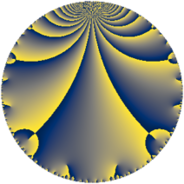# Properties

 Label 117.4.aLevel $117$ Weight $4$ Character orbit 117.a Rep. character $\chi_{117}(1,\cdot)$ Character field $\Q$ Dimension $15$ Newform subspaces $7$ Sturm bound $56$ Trace bound $2$

# Related objects

## Defining parameters

 Level: $$N$$ $$=$$ $$117 = 3^{2} \cdot 13$$ Weight: $$k$$ $$=$$ $$4$$ Character orbit: $$[\chi]$$ $$=$$ 117.a (trivial) Character field: $$\Q$$ Newform subspaces: $$7$$ Sturm bound: $$56$$ Trace bound: $$2$$ Distinguishing $$T_p$$: $$2$$

## Dimensions

The following table gives the dimensions of various subspaces of $$M_{4}(\Gamma_0(117))$$.

Total New Old
Modular forms 46 15 31
Cusp forms 38 15 23
Eisenstein series 8 0 8

The following table gives the dimensions of the cuspidal new subspaces with specified eigenvalues for the Atkin-Lehner operators and the Fricke involution.

$$3$$$$13$$FrickeDim.
$$+$$$$+$$$$+$$$$4$$
$$+$$$$-$$$$-$$$$2$$
$$-$$$$+$$$$-$$$$4$$
$$-$$$$-$$$$+$$$$5$$
Plus space$$+$$$$9$$
Minus space$$-$$$$6$$

## Trace form

 $$15 q + 82 q^{4} - 6 q^{5} + 2 q^{7} + O(q^{10})$$ $$15 q + 82 q^{4} - 6 q^{5} + 2 q^{7} - 54 q^{10} + 42 q^{11} - 13 q^{13} + 78 q^{14} + 218 q^{16} - 36 q^{17} - 162 q^{19} + 192 q^{20} - 16 q^{22} + 36 q^{23} + 635 q^{25} + 78 q^{26} + 888 q^{28} - 294 q^{29} - 730 q^{31} + 180 q^{32} - 184 q^{34} - 366 q^{35} + 78 q^{37} - 732 q^{38} - 1550 q^{40} - 402 q^{41} + 806 q^{43} + 600 q^{44} - 1996 q^{46} - 282 q^{47} - 91 q^{49} + 144 q^{50} - 624 q^{52} + 834 q^{53} + 452 q^{55} + 390 q^{56} - 1172 q^{58} + 1734 q^{59} + 1622 q^{61} + 48 q^{62} + 3626 q^{64} + 468 q^{65} + 2178 q^{67} - 2634 q^{68} - 3388 q^{70} + 1098 q^{71} - 1870 q^{73} - 3210 q^{74} - 1664 q^{76} - 1812 q^{77} - 1096 q^{79} + 1392 q^{80} - 168 q^{82} + 1422 q^{83} - 712 q^{85} - 5004 q^{86} - 456 q^{88} + 534 q^{89} - 676 q^{91} + 204 q^{92} + 5138 q^{94} + 3720 q^{95} + 1758 q^{97} + 5424 q^{98} + O(q^{100})$$

## Decomposition of $$S_{4}^{\mathrm{new}}(\Gamma_0(117))$$ into newform subspaces

Label Dim $A$ Field CM Traces A-L signs $q$-expansion
$a_{2}$ $a_{3}$ $a_{5}$ $a_{7}$ 3 13
117.4.a.a $1$ $6.903$ $$\Q$$ None $$0$$ $$0$$ $$12$$ $$2$$ $-$ $-$ $$q-8q^{4}+12q^{5}+2q^{7}+6^{2}q^{11}+13q^{13}+\cdots$$
117.4.a.b $1$ $6.903$ $$\Q$$ None $$5$$ $$0$$ $$7$$ $$-13$$ $-$ $-$ $$q+5q^{2}+17q^{4}+7q^{5}-13q^{7}+45q^{8}+\cdots$$
117.4.a.c $2$ $6.903$ $$\Q(\sqrt{14})$$ None $$-2$$ $$0$$ $$-24$$ $$0$$ $-$ $+$ $$q+(-1+\beta )q^{2}+(7-2\beta )q^{4}+(-12+\cdots)q^{5}+\cdots$$
117.4.a.d $2$ $6.903$ $$\Q(\sqrt{17})$$ None $$-1$$ $$0$$ $$3$$ $$-9$$ $-$ $+$ $$q-\beta q^{2}+(-4+\beta )q^{4}+(2-\beta )q^{5}+(-10+\cdots)q^{7}+\cdots$$
117.4.a.e $2$ $6.903$ $$\Q(\sqrt{7})$$ None $$0$$ $$0$$ $$0$$ $$-44$$ $+$ $-$ $$q+\beta q^{2}-q^{4}-4\beta q^{5}-22q^{7}-9\beta q^{8}+\cdots$$
117.4.a.f $3$ $6.903$ 3.3.3144.1 None $$-2$$ $$0$$ $$-4$$ $$30$$ $-$ $-$ $$q+(-1+\beta _{1})q^{2}+(3+\beta _{2})q^{4}+(-2+\cdots)q^{5}+\cdots$$
117.4.a.g $4$ $6.903$ 4.4.1520092.1 None $$0$$ $$0$$ $$0$$ $$36$$ $+$ $+$ $$q+\beta _{1}q^{2}+(14+\beta _{2})q^{4}-\beta _{3}q^{5}+(8+\cdots)q^{7}+\cdots$$

## Decomposition of $$S_{4}^{\mathrm{old}}(\Gamma_0(117))$$ into lower level spaces

$$S_{4}^{\mathrm{old}}(\Gamma_0(117)) \cong$$ $$S_{4}^{\mathrm{new}}(\Gamma_0(9))$$$$^{\oplus 2}$$$$\oplus$$$$S_{4}^{\mathrm{new}}(\Gamma_0(13))$$$$^{\oplus 3}$$$$\oplus$$$$S_{4}^{\mathrm{new}}(\Gamma_0(39))$$$$^{\oplus 2}$$